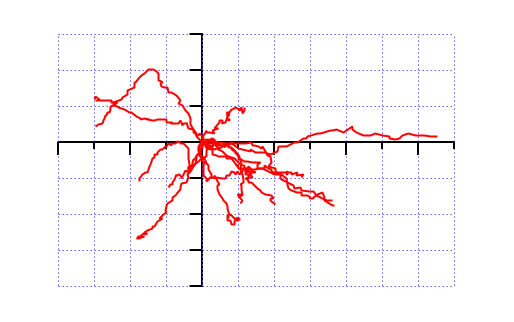# Round and Round

I thought I’d share a procedure for rotating a 2D set of coordinates about the origin. Why would you want do this? Well, we’ve been looking at cell migration in 2D – tracking nuclear position over time. Cells migrate at random and I previously blogged about ways to visualise these tracks more clearly. Part of this earlier procedure was to set the start of each track at (0,0). This gives a random hairball of tracks moving away from the origin. Wouldn’t it be a good idea to orient all the tracks so that the endpoint lies on the same axis? This would simplify the view and allow one to assess how ‘directional’ the cell tracks are. To rotate a set of coordinates, you need to use a rotation matrix. This allows you to convert the x,y coordinates to their new position x’,y’. This rotation is counter-clockwise.

$$x’ = x \cos \theta – y \sin \theta\,$$

$$y’ = x \sin \theta + y \cos \theta\,$$

However, we need to find theta first. To do this we need to find the angle between two lines, using this formula.

$$\cos \theta = \frac {\mathbf a \cdot \mathbf b}{\left \Vert {\mathbf a} \right \Vert \cdot \left \Vert {\mathbf b} \right \Vert}$$

The maths is kept to a minimum here. If you are interested, look at the code at the bottom.The two lines (a and b) are formed by the x-axis (origin to some point on the x-axis, i.e. y=0) and by a line running from the origin to the last coordinate in the series. This calculation can be done for each track with theta for each track being used to rotate the that whole track (x,y changed to x’,y’ for each point).

Here is an example of just a few tracks from an experiment. Typically we have hundreds of tracks for each experimental group and the code will blast through them all very quickly (<1 s).After rotation, the tracks are now aligned so that the last point is on the x-axis at y=0. This allows us to see how ‘directional’ the tracks are. The end points are now aligned, when they migrated there, how convoluted was their path.

The code to do this is up on Igor Exchange code snippets. A picture of the code is below (markup for code in WordPress is not very clear). See the code snippet if you want to use it.The weakness of this method is that acos (arccos) only gives results from 0 to Pi (0 to 180°). There is a correction in the procedure, but everything needs editing if you want to rotate the co-ordinates to some other plane. Feedback welcome.

Edit Jim Prouty and A.G. have suggested two modifications to the code. The first is to use complex waves rather than 2D real waves. Then use two native Igor functions r2polar or p2rect. The second suggestion is to use Matrix operations! As is often the case with Igor there are several ways of doing things. The method described here is long-winded compared to a MatrixOp and if the waves were huge these solutions would be much, much faster. As it is, our migration movies typically have 60 points and as mentioned rotator() blasts through them very quickly. More complex coordinate sets would need something more sophisticated.

The post title is taken from “Round & Round” by New Order from their Technique LP.